Function Repository Resource:

# PointsetDerivativeRoots

Get the roots of a derivative for applying the Lucas–Gauss theorem on a set of points

Contributed by: Ed Pegg Jr
 ResourceFunction["PointsetDerivativeRoots"][pts] applies the Lucas-Gauss derivative on a set of pts and finds the roots.

## Details

The Lucas–Gauss theorem states that the convex hull of the roots of any nonconstant complex polynomial contains the roots of its derivative.
With input {{r1,im1},{r2,im2},} and zi=ri+imi, the roots of derivative D[(z-z1)(z-z2),z] are returned as points.
Throughout this document, the roots of a derivative of a nonconstant complex polynomial generated by points are called "roots", out of convenience.

## Examples

### Basic Examples (2)

Find the roots of a set of points:

 In:=Out=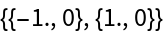By Marden's theorem, the roots of triangle vertices are the foci of an ellipse tangent to midpoints of the triangle's edges:

 In:=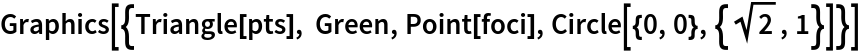Out=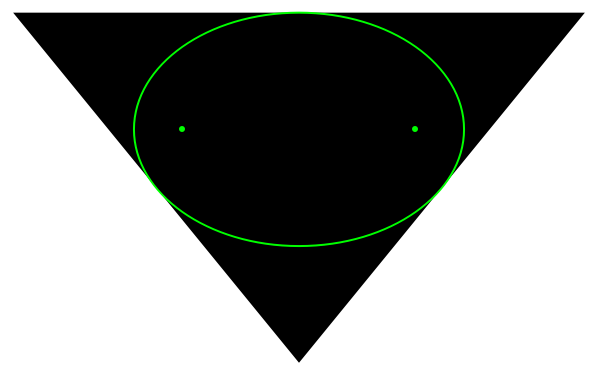Find the roots of a set of points:

 In:=Out=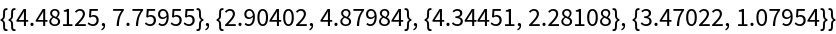By the Lucas–Gauss theorem, the convex hull of the roots is entirely contained by the convex hull of the original points:

 In:=Out=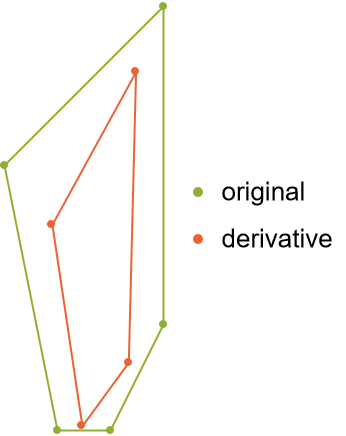In:=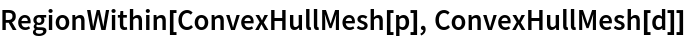Out=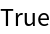### Scope (2)

The root of two points is the midpoint:

 In:=Out=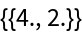The root of three points is given by the foci of the ellipse tangent to the midpoints of the triangle:

 In:=Out=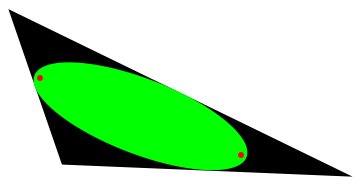This is equivalent to the Steiner circumellipse, scaled by a factor of 1/2:

 In:=Out=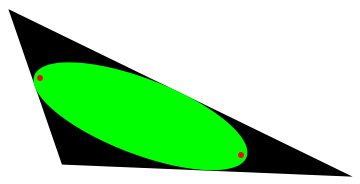### Possible Issues (1)

Much as in a convex hull, duplicating a point will not affect the result:

 In:=Out=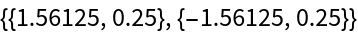### Neat Examples (1)

Show eleven levels of the Lucas-Gauss theorem:

 In:=Out=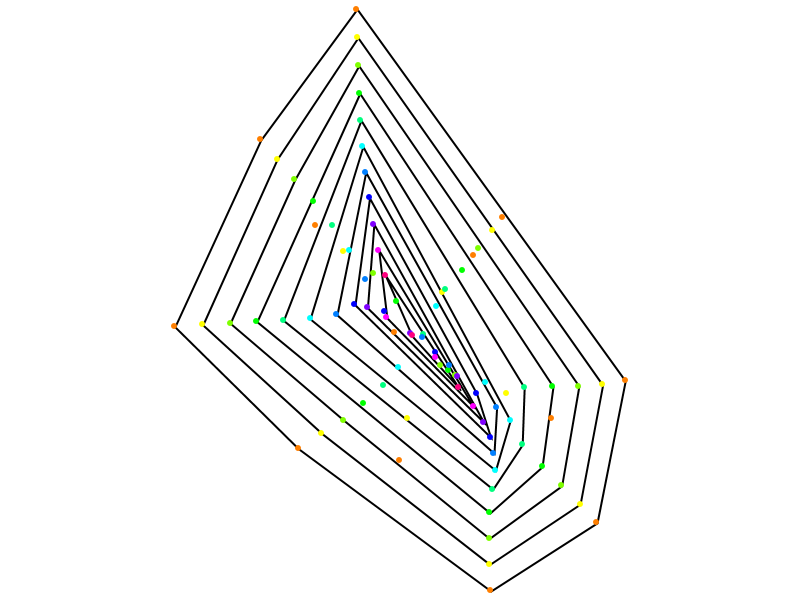## Version History

• 1.0.0 – 08 March 2023